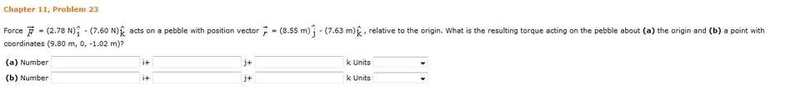# Find the Torque

## Homework StatementTorque = R x F

## The Attempt at a Solution

I'm only confused because we've only found the torque using the force and position vectors were given. When it says find the torque acting on the pebble about the origin and a point with coordinates (x, y, z) I'm not quite sure what to do to accomadate for this. I'm just fine with using the cross product, so can anyone help clarify what parts a and b are asking for? I'm pretty sure that part a is just telling me to find the torque using the vectors given without any changes. In part b I think that's telling me to use the new coordinates as the position vector. Can someone clarify this?

PhanthomJay
Homework Helper
Gold Member

## Homework StatementTorque = R x F

## The Attempt at a Solution

I'm only confused because we've only found the torque using the force and position vectors were given. When it says find the torque acting on the pebble about the origin and a point with coordinates (x, y, z) I'm not quite sure what to do to accomadate for this. I'm just fine with using the cross product, so can anyone help clarify what parts a and b are asking for? I'm pretty sure that part a is just telling me to find the torque using the vectors given without any changes. In part b I think that's telling me to use the new coordinates as the position vector. Can someone clarify this?
You are correct, the torque of a force has no meaning unless it is referenced to a coordinate or a mutual set of perpendicular axes that pass through the coordinate. In part b, the position vector runs from the new set of coordinates to the coordinates of the pebble. If you are familiar with the cross product manipulations, and can calculate the new position vector, then go for it. Personally, I prefer a more algebraic approach, where

Mx = Fyz + Fzy
My = Fxz + Fzx
Mz = Fxy + Fyx

where x, y, and z, are the perpendicular distances from the line of action of the appropriate force component to the new coordinate. Watch plus and minius signs. But it is what you are comfortable with that determines which method to use.